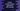# Get evenly spaced numbers in an interval using numpy linspace## Get evenly spaced numbers in an interval using numpy linspace:

numpy.linspace method is used to create an evenly spaced numbers in a given interval. This method is defined as like below:

``numpy.linspace(start, stop, num=50, endpoint=True, retstep=False, dtype=None, axis=0)``

Where,

• endpoint is an optional boolean value. It is True by default. If it is marked as True, stop is taken as the last sample. It is not included otherwise.
• start is an array_like value, the starting value of the sequence.
• stop is also an array_like value, the end value of the sequence. If endpoint is set to False, the sequence consists of all but the last of num + 1 evenly-spaced samples.
• num is the optional number of samples to generate. By default, it is 50 and it should be a non-negative value.
• retstep is an optional boolean value. If it is True, it returns (samples, step).
• dtype is an optional value, it is the type of the output array. If it is not given, the data type is inferred from start and stop.
• axis is an optional value. It is the axis in the result to store the value.

It returns an ndarray object, there will be num equally spaced samples. If the value of endpoint is True, these values will be in the closed interval [start, stop],

### Example of numpy linspace:

Let me show you an example of numpy linspace:

``````import numpy as np

print(np.linspace(4.0, 10.0, num=10))``````

It will print:

``````[ 4.          4.66666667  5.33333333  6.          6.66666667  7.33333333
8.          8.66666667  9.33333333 10.        ]``````

### Plotting using matplotlib:

Let’s use matplotlib library to plot the result of linspace on a graph:

``````import numpy as np
import matplotlib.pyplot as plt

x1 = np.linspace(0, 15, 10)
y1 = np.zeros(10)

plt.plot(x1, y1, '*')
plt.show()``````

It will give output as like below: